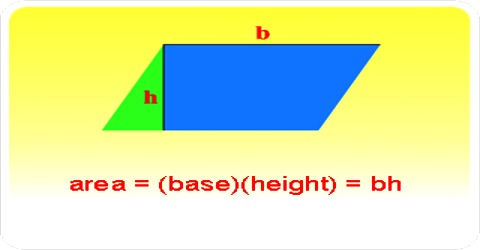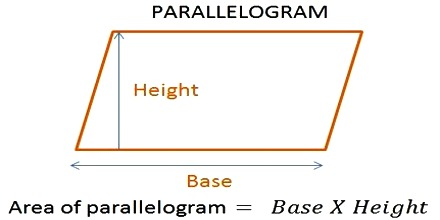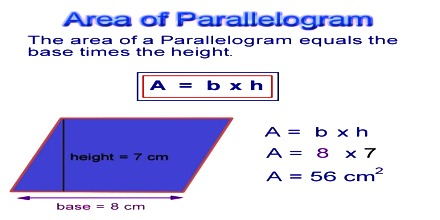Mathematic

# How to find the Area of a Parallelogram?Calculating the Area of a Parallelogram

The area of a parallelogram can be found by multiplying the base times the height. It means the number of square units it takes to completely fill a parallelogram.

Formula: Base × AltitudeIf a parallelogram has a base of length 6 inches and a height of 4 inches, its area is 6×4=24 square inches.

A parallelogram is a 4-sided shape formed by two pairs of parallel lines. Opposite sides are equal in length and opposite angles are equal in measure. To find the area of a parallelogram, multiply the base by the height. The formula is:

A = b × h

where, b is the base; and h is height.

Example:Find the area of a parallelogram with a base of 8 centimeters and a height of 7 centimeters.

Solution: A = b × h

A = (8 cm) × (7 cm) = 56 cm2.